This book is archived and will be removed July 6, 2022. Please use the updated version.

Process Costing

# 30 Explain and Compute Equivalent Units and Total Cost of Production in a Subsequent Processing Stage

In many production departments, units are typically transferred from the initial stage to the next stage in the process. When the units are transferred, the accumulated cost per unit is transferred along with them. Since the unit being produced includes work from all of the prior departments, the transferred-in cost is the cost of the work performed in all earlier departments.

When the hickory size 5A drumsticks have completed the shaping process, they are transferred to the packaging department along with the inventory costs of \$29,775. The inventory costs of \$29,775 were \$8,775 for materials and \$21,000 for conversion costs and were calculated in (Figure). During the month of July, Rock City Percussion purchased raw material inventory of \$2,000 for the packaging department. As with the shaping department, the packaging department tracks its costs and requisitions the raw material from the material storeroom. The packaging department has computed direct material costs of \$2,000, direct labor costs of \$13,000, and applied overhead of \$9,100, for a total of \$22,100 in conversion costs. Equivalent units are computed for this department, and a new cost per unit is computed.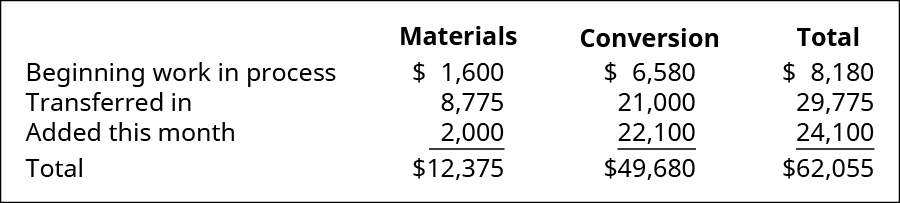As with calculating the equivalent units and total cost of production in the initial processing stage, there are four steps for calculating these costs in a subsequent processing stage.

### Step One: Determining the Stage 2 Units to Which Costs Will Be Assigned

In the initial manufacturing department, there is beginning inventory, and units are started in production. In subsequent stages, instead of starting new units, units are transferred in from the prior department, but the accounting process is the same. Returning to the example, Rock City Percussion had a beginning inventory of 750 units in the packaging department. When the 7,500 sticks are transferred into the packaging department from the shaping department, the total number of units to account for in the reconciliation is 8,250, which is the total of the beginning WIP and the units transferred in: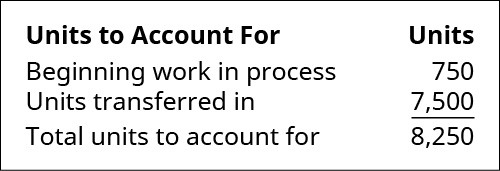The reconciliation of units to account for are the same for each department. The units that were completed and transferred out plus the ending inventory equal the total units to account for. The packaging department for Rock City Percussion completed 6,500 units and transferred them into finished goods inventory. Since the maximum number of units to possibly be completed is 8,250 and no units were lost to spoilage, the number of units in the packaging department’s ending inventory must be 1,750. The total of the 6,500 units completed and transferred out and the 1,750 units in ending inventory equal the 8,250 possible units in the packaging department.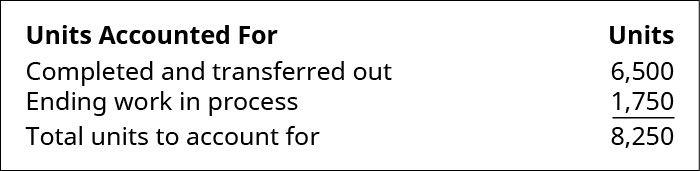### Step Two: Computing the Stage 2 Equivalent Units of Production

The only direct material added in the packaging department for the 5A sticks is packaging. The packaging materials are added at the beginning of the process, so all the materials have been added before the units are transferred out, but all of the conversion elements have not. As a result, the number of equivalent units for material costs and for conversion costs remaining in ending inventory is different for the testing and sorting department. As you’ve learned, all of the units transferred to the next department must be 100% complete with regard to that department’s cost, or they would not be transferred. The process cost system must calculate the equivalent units of production for units completed (with respect to materials and conversion) and for ending WIP with respect to materials and conversion.

For the packaging department, the materials are 100% complete with regard to materials costs and 40% complete with regard to conversion costs. The 6,500 units completed and transferred out to the finishing department must be 100% complete with regard to materials and conversion, so they make up 6,500 (6,500 × 100%) units. The 1,750 ending WIP units are 100% complete with regard to material and have 1,750 (1,750 × 100%) equivalent units for material. The 1,750 ending WIP units are only 40% complete with regard to conversion costs and represent 700 (1,750 × 40%) equivalent units.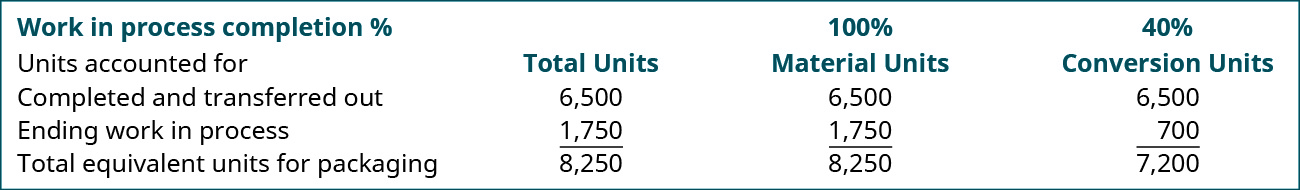### Step Three: Determining the Stage 2 Cost per Equivalent Unit

Once the equivalent units for materials and conversion are known for the packaging department, the cost per equivalent unit is computed in a manner similar to the calculation for the units accounted for. The costs for material and conversion need to reconcile with the department’s beginning inventory and the costs incurred for the department during that month.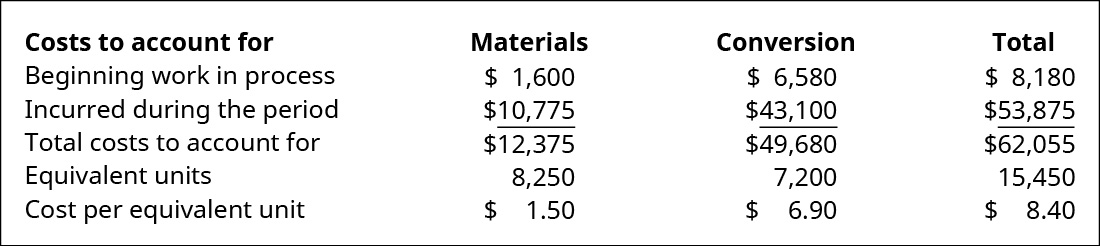The total materials costs for the period (including any beginning inventory costs) are computed and divided by the equivalent units for materials. The same process is then completed for the total conversion costs. The total of the cost per unit for materials (\$1.50) and for conversion costs (\$6.90) is the total cost of each unit transferred to the testing and sorting department.

### Step Four: Allocating the Costs to the Units in the Finishing Department

Now you can determine the cost of the units transferred out and the cost of the units still in process in the finishing department. For the goods transferred out, simply take the units transferred out times the sum of the two equivalent unit costs (materials and conversion) because all items transferred to the next department are complete with respect to materials and conversion, so each unit brings all its costs. But the ending WIP value is determined by taking the product of the work in process materials units and the cost per equivalent unit for materials plus the product of the work in process conversion units and the cost per equivalent unit for conversion.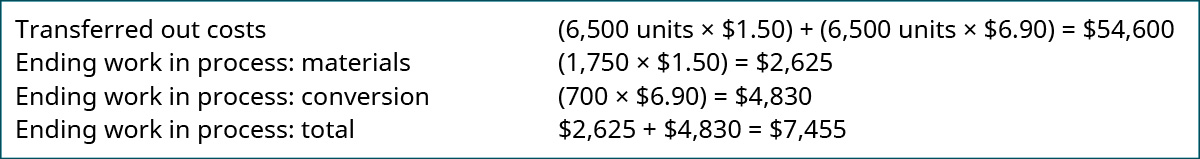### Key Concepts and Summary

• The total units to account for is the number of units in the beginning work in process inventory plus the number of units transferred from the prior department; this total also represents the number of units completed plus the number of units in the ending work in process inventory.
• The cost per equivalent units for materials is the total of the material costs for the beginning work in process inventory plus the cost of material transferred in to the department plus the total of material costs incurred during the period.
• The cost per equivalent unit for conversion costs is the total of the conversion costs for the beginning work in process inventory plus the conversion costs transferred in plus the total of conversion costs incurred during the period.

(Figure)The costs to be accounted for consist of which of the following?

1. costs added during the period
2. costs of the units in ending inventory
3. costs started and transferred during the period
4. costs in the beginning inventory and costs added during the period

(Figure)Which of the following is the step in which materials, labor, and overhead are detailed?

1. determining the units to which costs are assigned
2. determining the equivalent units of production
3. determining the cost per equivalent units
4. allocating the costs to the units transferred out and the units partially completed

C

(Figure)How does process costing treat the costs transferred in from another department?

The costs transferred in are treated in the same way as direct material that is added to production at the beginning of the process.

(Figure)Why does each department have its own work in process inventory?

(Figure)A production department within a company received materials of \$10,000 and conversion costs of \$10,000 from the prior department. It added material of \$27,200 and conversion costs of \$53,000. The equivalent units are 20,000 for material and 18,000 for conversion. What is the unit cost for materials and conversion?

(Figure)Production data show 35,920 units were transferred out of a stage of production and 6,150 units remained in ending WIP inventory that was 100% complete to material and 35% complete to conversion. The unit material cost is \$5 for material and \$8 for conversion. What is the amount of inventory transferred out and remaining in ending work in process inventory?

(Figure)A production department within a company received materials of \$7,000 and conversion costs of \$5,000 from the prior department. It added material of \$78,400 and conversion costs of \$47,000. The equivalent units are 5,000 for material and 4,000 for conversion. What is the unit cost for materials and conversion?

(Figure)Production data show 15,200 units were transferred out of a stage of production and 3,500 units remained in ending WIP inventory that was 100% complete to material and 60% complete to conversion. The unit material cost is \$9 for material and \$4 for conversion. What is the amount of inventory transferred out and remaining in ending work in process inventory?

(Figure)The finishing department started the month with 700 units in WIP inventory. It received 2,200 units from the molding department and transferred out 2,150 units. How many units were in process at the end of the month?

(Figure)The packaging department began the month with 500 units that were 100% complete with regard to material and 85% complete with regard to conversion. It received 9,500 units from the processing department and ended the month with 750 units that were 100% complete with regard to materials and 30% complete with regard to conversion. With a \$5 per unit cost for conversion and a \$5 per unit cost for materials, what is the cost of the units transferred out and remaining in ending inventory?

(Figure)Production information shows these costs and units for the smoothing department in August.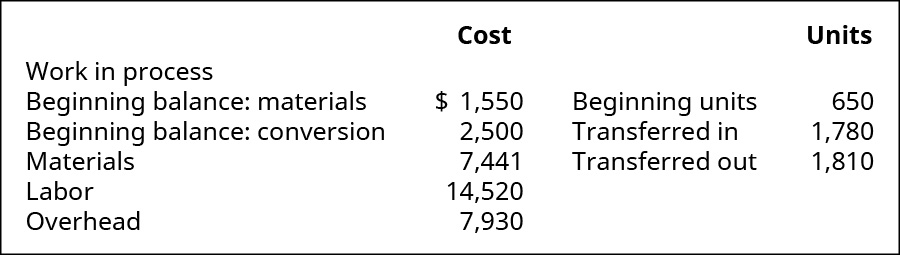All materials are added at the beginning of the period. The ending work in process is 30% complete as to conversion. What is the value of the inventory transferred to finished goods and the value of the WIP inventory at the end of the month?

(Figure)Given the following information, prepare a production report with materials added at the beginning and ending work in process inventory being 25% complete with regard to conversion costs.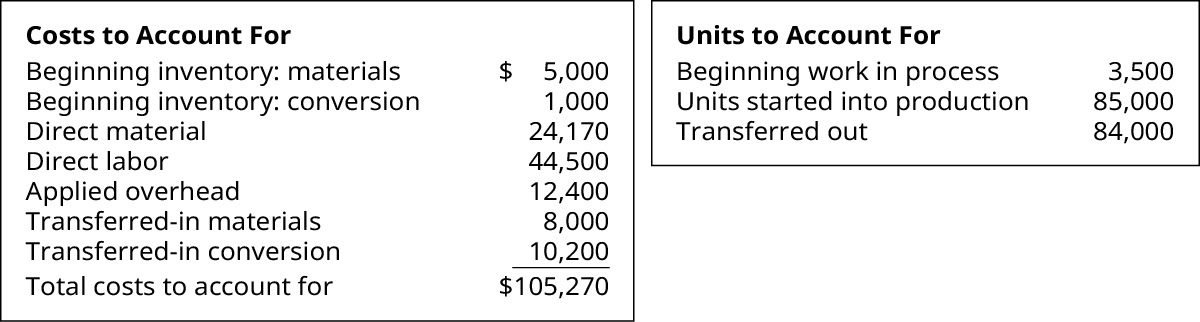(Figure)Complete this production cost report: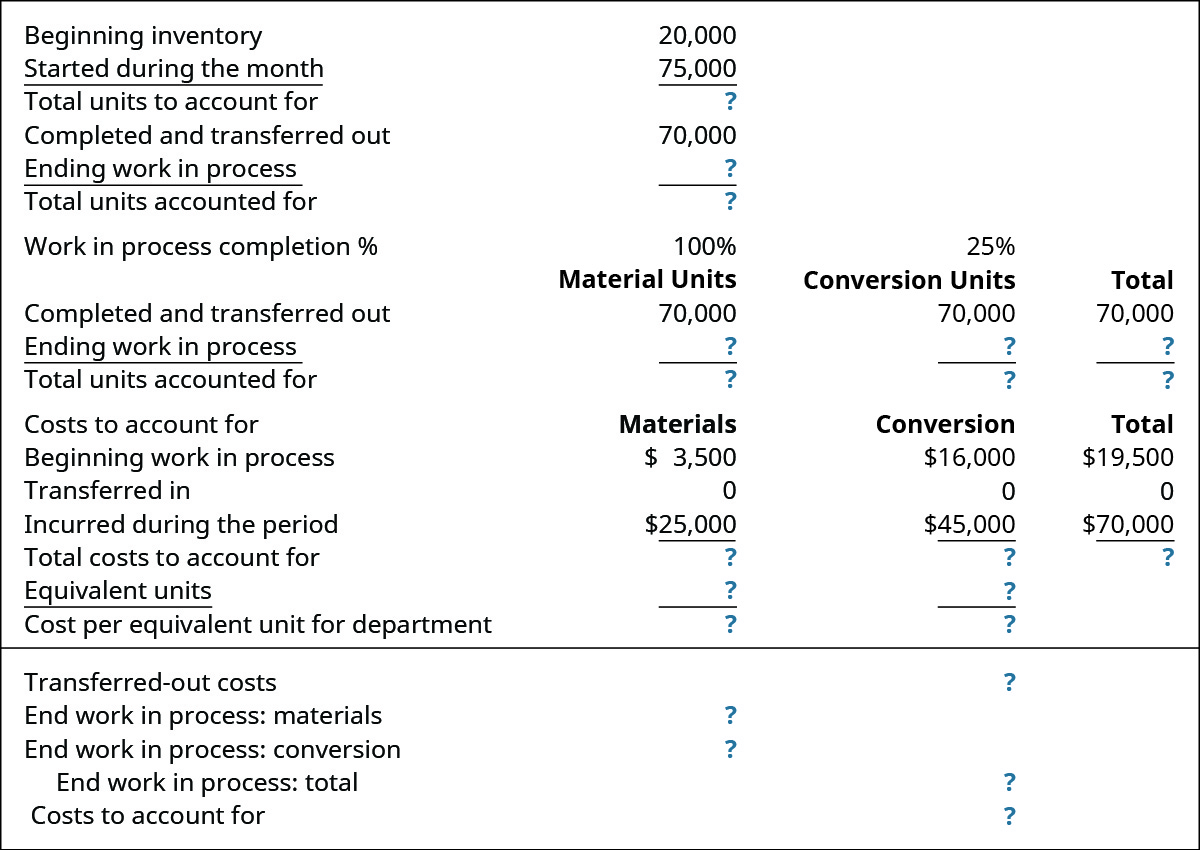(Figure)The finishing department started the month with 600 units in WIP inventory. It received 1,500 units from the molding department and ended the month with 550 units still in process. How many units were transferred out?

(Figure)The packaging department began the month with 750 units that were 100% complete with regard to material and 25% complete with regard to conversion. It received 9,500 units from the processing department and ended the month with 500 units that were 100% complete with regard to materials and 75% complete with regard to conversion. With a \$7 per unit material cost and a \$4 per unit cost for conversion, what is the cost of the units transferred out and remaining in ending inventory?

(Figure)Production information shows these costs and units for the smoothing department in August.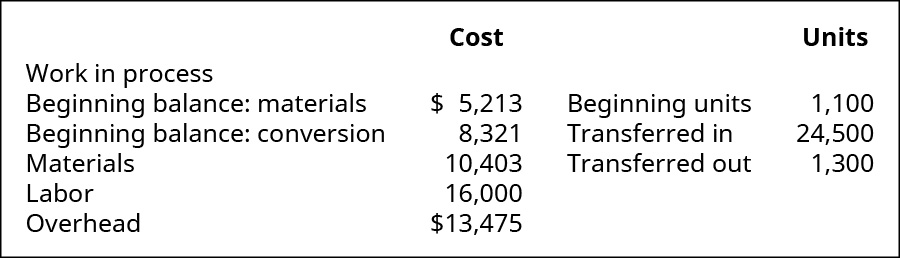What is the value of the inventory transferred out to finished goods and the value of the WIP inventory at the end of the month, assuming conversion costs are 30% complete?

(Figure)Given the following information, prepare a production report with materials added at the beginning and ending work in process inventory being 80% complete with regard to conversion costs.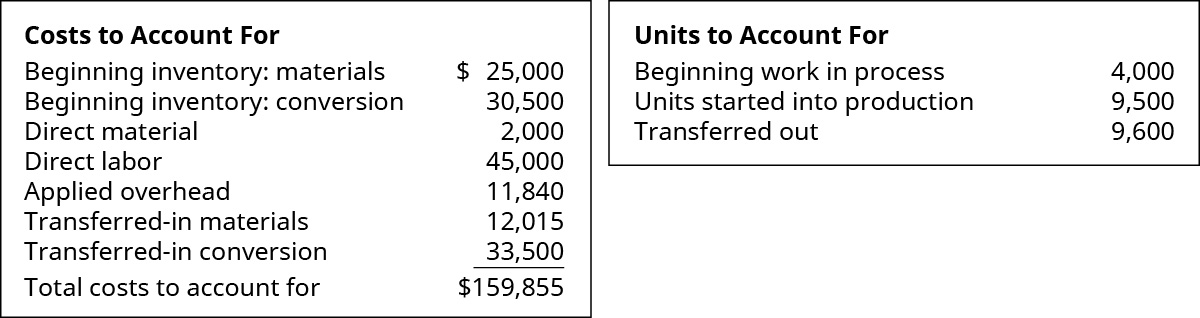(Figure)How is process costing for a single manufacturing department different from a manufacturing company with multiple departments?